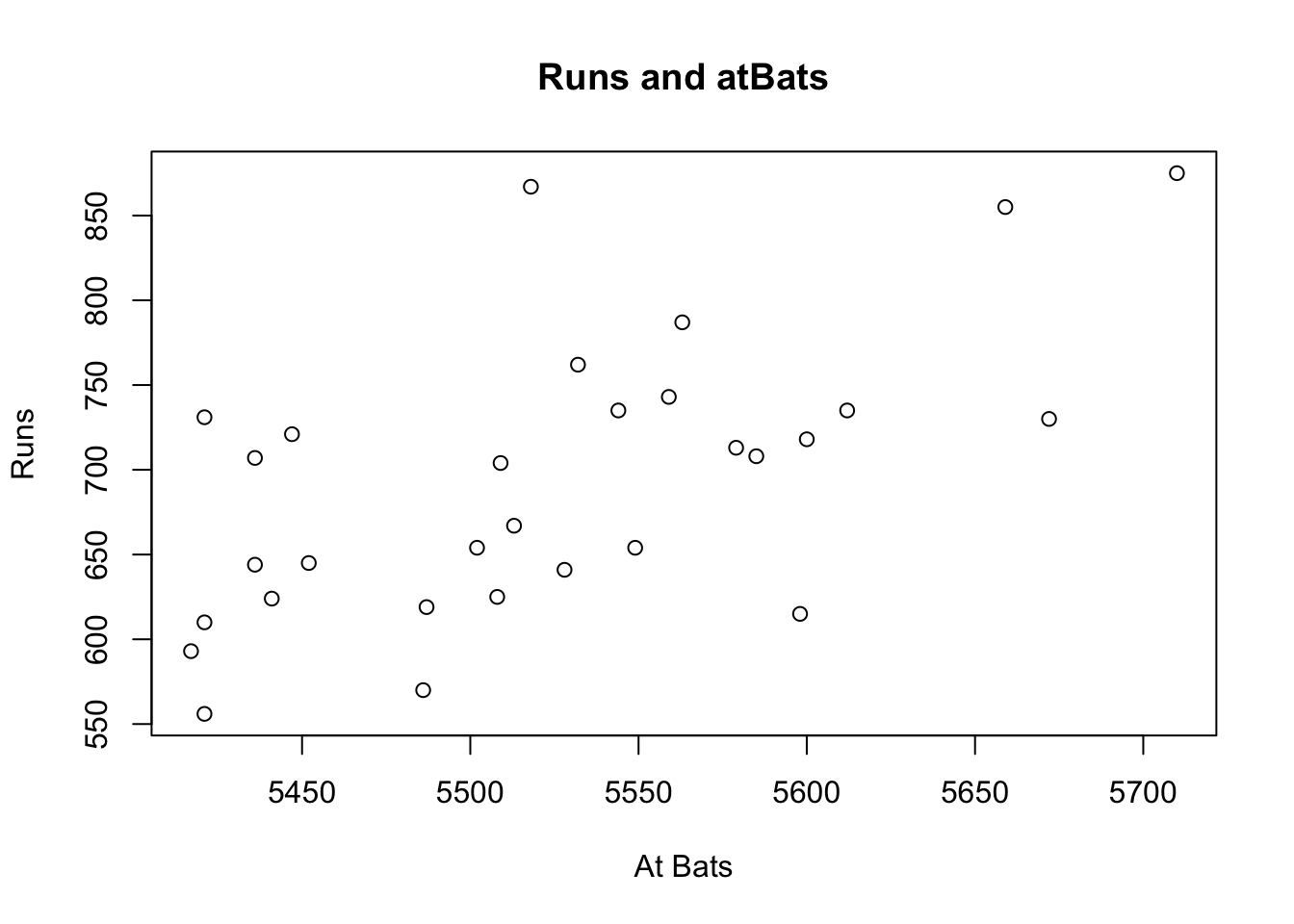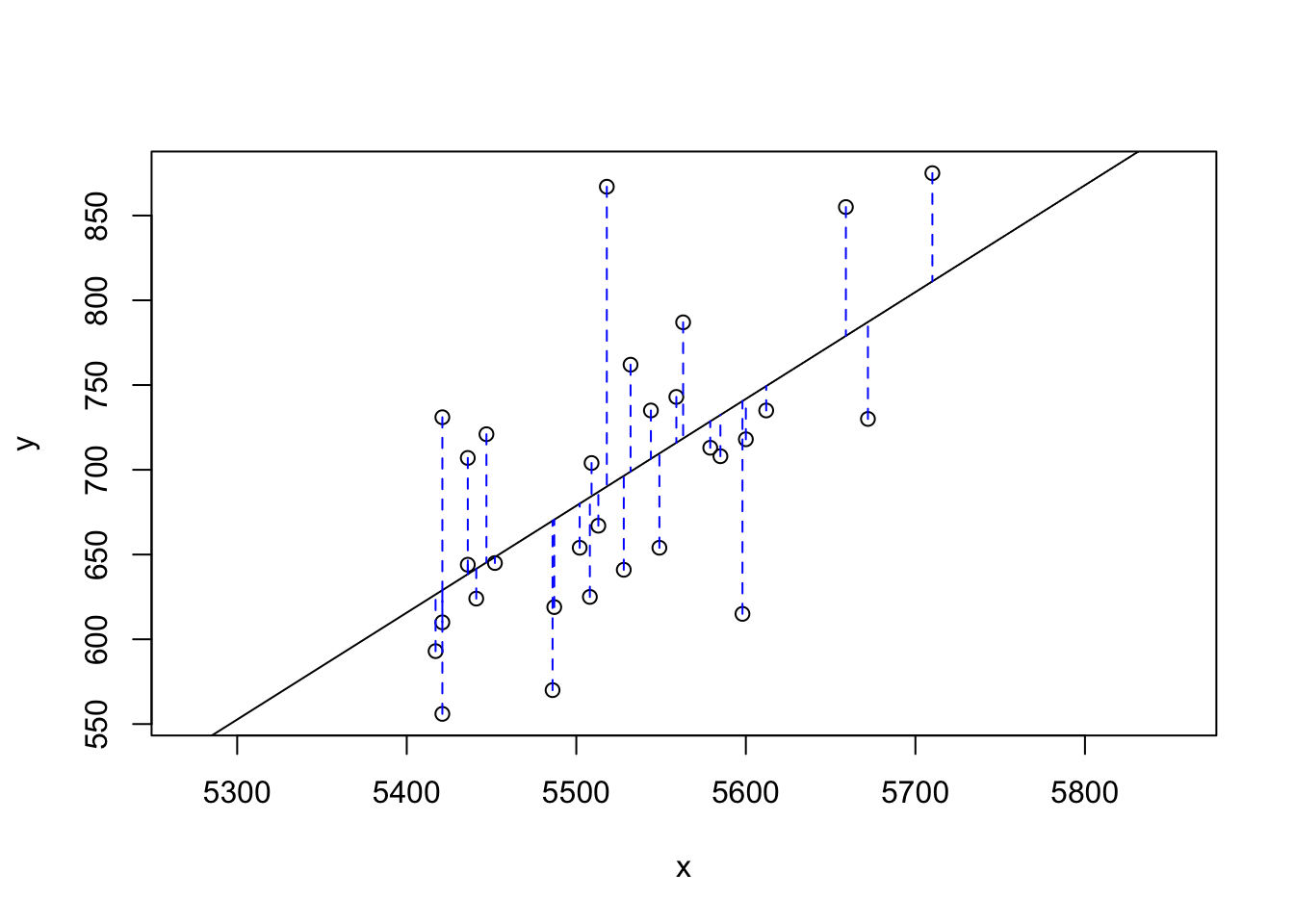## Batter up

The movie Moneyball focuses on the “quest for the secret of success in baseball”. It follows a low-budget team, the Oakland Athletics, who believed that underused statistics, such as a player’s ability to get on base, better predict the ability to score runs than typical statistics like home runs, RBIs (runs batted in), and batting average. Obtaining players who excelled in these underused statistics turned out to be much more affordable for the team.

In this lab we’ll be looking at data from all 30 Major League Baseball teams and examining the linear relationship between runs scored in a season and a number of other player statistics. Our aim will be to summarize these relationships both graphically and numerically in order to find which variable, if any, helps us best predict a team’s runs scored in a season.

## The data

Let’s load up the data for the 2011 season.

load("more/mlb11.RData")

In addition to runs scored, there are seven traditionally used variables in the data set: at-bats, hits, home runs, batting average, strikeouts, stolen bases, and wins. There are also three newer variables: on-base percentage, slugging percentage, and on-base plus slugging. For the first portion of the analysis we’ll consider the seven traditional variables. At the end of the lab, you’ll work with the newer variables on your own.

1. What type of plot would you use to display the relationship between runs and one of the other numerical variables? Plot this relationship using the variable at_bats as the predictor. Does the relationship look linear? If you knew a team’s at_bats, would you be comfortable using a linear model to predict the number of runs?

Answer: Scatterplot can be used to display the relationship between these 2 variables.

plot(mlb11$runs ~ mlb11$at_bats, main = "Runs and atBats", xlab = "At Bats", ylab = "Runs")Answer (cont.): The relationship looks somewhat linear even though correlation doesnt appear very strong. I would feel comfortable using linear model to predict the number of runs.

If the relationship looks linear, we can quantify the strength of the relationship with the correlation coefficient.

cor(mlb11$runs, mlb11$at_bats)
##  0.610627

## Sum of squared residuals

Think back to the way that we described the distribution of a single variable. Recall that we discussed characteristics such as center, spread, and shape. It’s also useful to be able to describe the relationship of two numerical variables, such as runs and at_bats above.

1. Looking at your plot from the previous exercise, describe the relationship between these two variables. Make sure to discuss the form, direction, and strength of the relationship as well as any unusual observations.

Answer: There seams to be a positive correlation between the 2 variables, it is not very strong and there are some outliers.

Just as we used the mean and standard deviation to summarize a single variable, we can summarize the relationship between these two variables by finding the line that best follows their association. Use the following interactive function to select the line that you think does the best job of going through the cloud of points.

plot_ss(x = mlb11$at_bats, y = mlb11$runs)## Click two points to make a line.

## Call:
## lm(formula = y ~ x, data = pts)
##
## Coefficients:
## (Intercept)            x
##  -2789.2429       0.6305
##
## Sum of Squares:  123721.9

After running this command, you’ll be prompted to click two points on the plot to define a line. Once you’ve done that, the line you specified will be shown in black and the residuals in blue. Note that there are 30 residuals, one for each of the 30 observations. Recall that the residuals are the difference between the observed values and the values predicted by the line:

$e_i = y_i - \hat{y}_i$

The most common way to do linear regression is to select the line that minimizes the sum of squared residuals. To visualize the squared residuals, you can rerun the plot command and add the argument showSquares = TRUE.

plot_ss(x = mlb11$at_bats, y = mlb11$runs, showSquares = TRUE)